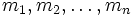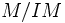Nakayama's lemma

View other indispensable lemmata

Statement

Let$R$ be a commutative unital ring, and$I$ be an ideal contained inside the Jacobson radical of$R$. Let$M$ be a finitely generated$R$-module. Then the following are true:

1. If$IM = M$ then$M = 0$
2. If$N$ is a submodule of$M$ such that$N + IM = M$, then$N = M$
3. If$m_1, m_2, \ldots, m_n$ have images in$M/IM$ that generate it as a$R$-module, then$m_1, m_2, \ldots, m_n$ generate$M$ as a$R$-module

In the particular case where$R$ is a local ring, the Jacobson radical is the unique maximal ideal in$R$.

Related facts

The graded Nakayama's lemma is a related fact true for graded rings.